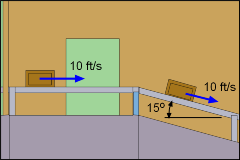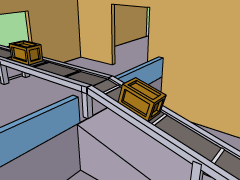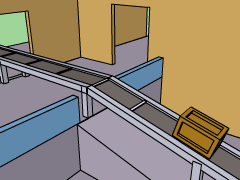Ch 2. Particle Force and Acceleration Multimedia Engineering Dynamics Rect.Coord. Normal/Tang. Coord. PolarCoord. Orbital Mechanics Computational Mechanics
 Chapter - Particle - 1. General Motion 2. Force & Accel. 3. Energy 4. Momentum - Rigid Body - 5. General Motion 6. Force & Accel. 7. Energy 8. Momentum 9. 3-D Motion 10. Vibrations Appendix Basic Math Units Basic Equations Sections Search eBooks Dynamics Fluids Math Mechanics Statics Thermodynamics Author(s): Kurt Gramoll ©Kurt GramollDYNAMICS - CASE STUDY IntroductionProblem Graphic At the ACME factory, crates containing precious goods are carried through the plant on conveyor belts. When a conveyor belt stops, the boxes must not slide or the goods may be damaged. What is known: The conveyor belts move at a constant speed of 10 ft/s. The crates first travel on a horizontal belt, then down a belt with a 15o decline. The static, μs, and kinetic (dynamic), μk, coefficient of friction, μs, between the belt and the crates are = 0.5 and 0.3, respectively.Box Stops Questions What is the shortest distance in which the horizontal belt can be brought to a stop without causing the crates to slide? What is the shortest distance for the inclined belt?Boxes Slides Approach Using Newton's Second Law, relate the forces acting on the crate with its acceleration. Analyze the problem in terms of rectangular coordinates. Since no sliding actually occurs, use the static coefficient of friction. Derive an equation for the position by integrating the acceleration equation a = d2x/dt2.

Practice Homework and Test problems now available in the 'Eng Dynamics' mobile app
Includes over 400 problems with complete detailed solutions.
Available now at the Google Play Store and Apple App Store.